# 1st PUC Computer Science Previous Year Question Paper March 2015 (South)

Students can Download 1st PUC Computer Science Previous Year Question Paper March 2015 (South), Karnataka 1st PUC Computer Science Model Question Papers with Answers helps you to revise the complete Karnataka State Board Syllabus and score more marks in your examinations.

## Karnataka 1st PUC Computer Science Previous Year Question Paper March 2015 (South)

Time: 3:15 Hours
Max. Marks: 100

PART – A

I. Answer all the following questions. Each question carries one mark. (10 × 1 = 10)

Question 1.
Who is the father of Computer?
The father of computer is Charles Babbage.

Question 2.
Expand the term MOUSE.
Mechanically Operated User Serial Engine.

Question 3.
What is an algorithm?
An algorithm is a “step by step procedure to solve a given problem infinite number of steps”.

Question 4.
Give the difference between I++ and ++I.
The I++ is a post-increment and ++I is pre-increment unary operator.Question 5.
What does cout stand for?
The cout stand for console output.

Question 6.
Name the post tested loop.
The post tested loop is WHILE-DO control structure.

Question 7.
What is an array.
Array is a group of similar elements that share a common name.

Question 8.
Define function.
A FUNCTION is a self contained block of statements that perform a specific task with a name given to it.Question 9.
What is a word processor?
Word Processor is an application Software that enables you to create, edit, print and save documents for future retrieval and reference.

Question 10.
List the types of charts in ESS.
The different chart types in ESS are Bar chart, Pie chart, Column chart, etc.,

PART – B

II. Answer any. Five of the following questions. Each question carries two marks: (5 × 2 = 10)

Question 11.
Explain hybrid computer.
Computers which combine features of both analog and digital operating principle are called hybrid computers.

Question 12.
Give any two input devices.
The two input devices are

1. Keyboard
2. Mouse.Question 13.
Write any two functions of operating system.
The two functions of operating system are process management, memory management and translation service etc.,

Question 14.
Give two differences between while and do-while loop.

1. The while loop is called as pre-tested loop and do-while loop is called as post-tested loop.
2. The while loop test the condition in the beginning of the loop structure. Whereas, do-while test the condition at the end of the body of loop structure.

Question 15.
Define inheritance.
Inheritance is the process by which objects can acquire the properties of objects of other class.

Question 16.
Name the different types of type conversion.
The different types of type conversion are

• Implicit conversion and
• Explicit conversion.Question 17.
Mention the different types of functions.
The two types of functions are in-built functions and user defined functions. The functions are also classified as function with no argument and no return value, function with argument and no return value, function with argument and return value and recursive function.

Question 18.
What is the use of word art?
The word art is application of text that can be presented as graphical rich text. The graphical text can be filled with effects, shown upside down, wavy texts, text in arch shape etc.,

PART – C

III. Answer any Five of the following Questions. Each question carries three marks: (5 × 3 = 15)

Question 19.
Explain impact printers. Give an example.
Impact printers forms characters or images by striking a mechanism such as a print hammer or wheel against an inked ribbon, leaving an image on paper. For example, dot matrix printer.

Question 20.
Convert 11001010(2) to decimal, octal.128 + 64 + 8 + 2 = 202(10)
11001010(2) make a set of 3 bits and then find its decimal number.Question 21.
What is the use of language translator? Explain.
The language translators translate user written high level language programs to equivalent machine or assembly language program. There are three different types of translators. They are compiler, interpreter and assembler.

1. Compiler:
It is a computer language translator that checks the entire user-written program and if error-free, produces a complete program in machine language.

2. Interpreter:
The Interpreter is a language translator which translates high level programs one statement at a time and, if error free, executes.Question 22.
Mention three advantages of flow chart.
The three Advantages of flowchart are:

1. It is a means of communication and easy to understand
2. Easy to convert into a program code.
3. Independent of programming language. i.e., a flowchart can be used to write programs using different programming languages.
4. Easy to test the program for errors and easy removal of such errors.

Question 23.
Write the general structure of C++ program.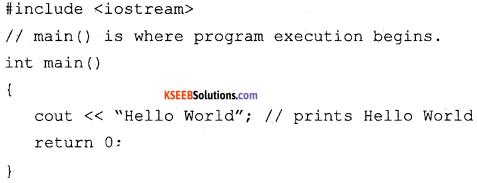The various parts of the above program:
1. headers, which contain information that is either necessary or useful to program. For this program, the header is needed.

2. The next line // main() is where program execution begins, is a single-line comment available in C++. Single-line comments begin with // and stop at the end of the line.

3. The line int main() is the main function where program execution begins.

4. The pair of { } indicates the body of the main function.

5. The next line cout<< “This is my first C++ program.”; causes the message “This is my first C++ program” to be displayed on the screen.

6. The next line return 0; terminates main( )function and causes it to return the value 0 to the calling process.

Question 24.
Explain sector() with an example.
It is a wrong question.Question 25.
Write the syntax and example of 2-dimensional array declaration.
Declaration syntax of two dimensional array.
Syntax:
<data-type> <array-name> [<rowsize>] [<columnsize>];
Example: int marks;

Question 26.
What is a structure? Give an example.
A structure is a collection of variable which can be same or different types.
Example: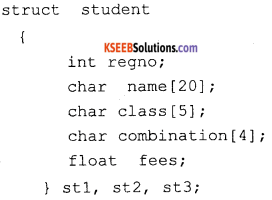PART – D

IV. Answer any Seven of the following questions. Each question carries Five marks: (7 × 5 = 35)

Question 27.
Draw the neat block diagram of a computer and explain.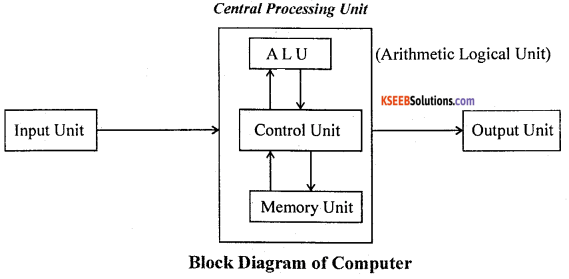1. Input Unit:
It is a device through which we enter the program and data into the computer. The data is then stored and processed with the help of programs.

2. Central Processing Unit:
The Central Processing Unit (CPU) is divided into three units namely, the control unit, the arithmetic, and logic unit and the memory unit.

3. Control Unit:
This unit is considered to be the nervous system of the computer. It controls all arithmetic operations to be performed. It also coordinates the functions of all hardware units of the computer.

4. Arithmetic and Logic Unit (AL U):
This unit performs all arithmetic calculations and logical operations involved in the program.

5. Memory Unit:
The function of this unit is to store the data and the set of instructions given by the programmer. The computation results are stored in the memory and they may be used for subsequent computations or sent to the output unit.

6. Output Devices:
Output devices receive information from the CPU and present it to the user in the desired form. Some common output devices are visual display unit (VDU), Printer, etc.,

Question 28.
Subtract 28(10) from 14(10) using 2’s complement.
14 – 28 = -14.
14(10) = 01110(2), 29(10) = 11100(2)
1’s complement of 11100 is 00011
2’s complement is 00011 + 1 = 00100
Now add, minuend with subtrahend, i.e.,01110 + 00100= 10010
Find the 1’s complement of 10010 is 01101 and
2’s complement of 01101 is 01101 + 1 = 1110(1).

Question 29.
Write an algorithm to find largest number in a input three numbers.

• Step 1: start.
• Step 2: input a, b,c
• Step 3: let Max=a .
• Step 4: is b > Max? then
• Max = b
• Step 5: is c > max? then
• Max = c
• Step 6: print Max
• Step 7: stopQuestion 30.
What is an expression? Explain the types of expression.
An expression is a combination of variables, constants and operators written according to the syntax of C++ language. In C++ every expression evaluates to a value i.e., every expression results in some value of a certain type that can be assigned to a variable.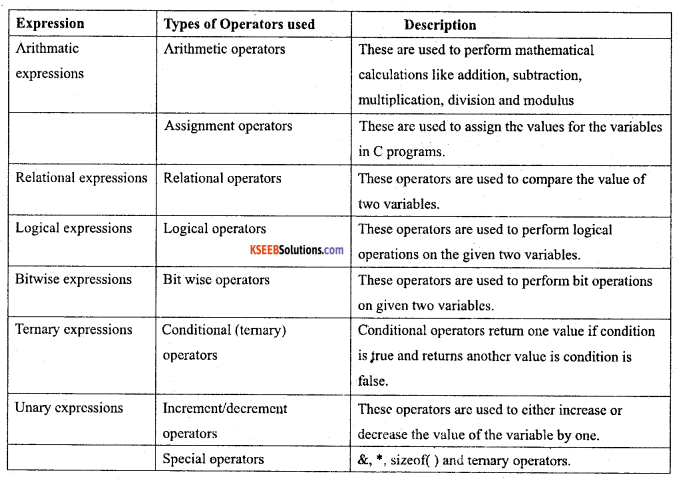Question 31.
Write a program to find the area and circumference of a circle.Question 32.
Briefly explain the working of switch statement with an example.
Switch statement compares the value of an expression against a list of integers or character constants. The list of constants are listed using the “case” statement along with a “break” statement to end the execution.
Example: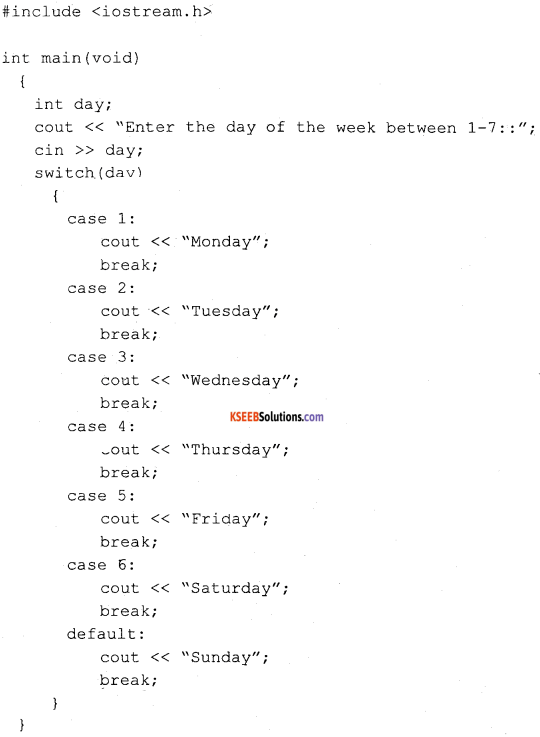Result:
Enter the day of the week between 1 – 7 :: 7
Sunday

In the above Control Structure example the “switch” statement is used to find the day of the week from the integer input got from the user. The value present in the day is compared for equality with constants written in the word case.

Since no equality is achieved in the above example (from 1 to 6) as they’entered value is 7, default is selected and gives “Sunday” as a result.Question 33.
Explain five string handling functions with syntax and examples.
1. Strlen( ) function:

• It calculates the length of the given string and returns the number of characters in a string.
• Syntax:

integer variable = strlen(string);

• Ex: length = strlen(“Bharath”);
• Output : 7

2. Strcat() Function:

• It adds the characters of one string to the end of another string. It is used to join the two strings together.
• Syntax:

strcat(stringl, string2);

• Ex: string1 = ”Bharath” string2 = ”Bhushan”, strcat(stringl, string2);
• Output: Bharath Bhushan

3. Strrev() Function:

• It reverses the characters in a string.
• Syntax:

strrev(string); .

• Ex: strrev(“ABCD”);
• Output: DCBA

4. Strlwr() Function

• Converts all characters in a string to lower (small letters) case.
• Syntax:

strlwr(string);

• Ex: strlwr(“BHARATH”)
• Output: bharath

5. Strupr() Function

• Converts all characters in a string to upper (capital letters) case.
• Syntax:

strupr(string);

• Ex: strupr(“bharath”)
• Output: BHARATH

Question 34.
Discuss the following terms.

1. Function prototype
2. Actual arguments
3. formal arguments
4. local variable
5. Global variable.

1. Function prototype:
The declaration of a function before it is used or called is known as function prototype. Ex: int demo (int, int, int);

2. Actual arguments:
Actual parameters: the data that is passed by the calling function as arguments/parameters is known as actual arguments, i.e., the arguments which are present at the time of function call.

3. Formal arguments:
Formal parameters: are the names of the argument/parameter in the function header of called function. Formal parameter values are used by the called function body.

4. Local (internal) Variables:
The variables declared within function block are called local variables. A variable can be used only within the function where it is defined in. Such a variable is accessible only from the function or block in which it is declared.

5. Global Variables:
They are the variables that are declared outside of main( ) function body or any other functional block. These variables can be used by all modules and functions in the program.Question 35.
Explain any five mathematical functions in MS excel with example.
1. abs() function in excel returns the absolute value of a number. The absolute value of a number is the number without its sign.
For example, Abs(-25) output: 25

2. Fact() function returns the factorial of a number. The factorial of a number is equal to 1*2*3*…* number.
For example, fact(5) output: 120

3. The mod() function returns the remainder after number is divided by divisor. The result has the same sign as divisor.
MOD(number, divisor)
For example, Mod(5,2) output: 1

4. The power () function returns the result of a number raised to a power.
POWER(number, power)
For example, power(5,2) output: 25

The sum() function adds all the numbers that is specified in the brackets. For example, SUM(A1 : A5) adds all the numbers that are contained in cells A1 through A5. For another example, SUM(A1, A3, A5) adds the numbers that are contained in cells A1, A3, and A5.

Question 36.
Mention different features of MS excel.
The different features of MS excel is as follows;
1. Excel Charts:
Excel provides a vast number of chart types and options.

2. Formulas and Functions:
Another great feature of Excel is the ability to embed formulas into cells to calculate data. In addition, an impressive list of built-in functions is provided that can be used to summarize data.

3. Cell Formatting:
You can customize the formatting of a cell or range of cells. You can apply borders, cell background color, font size and color, number formatting and much more.

4. Sorting and Filtering:
You can reorder the data or pick out just the data you need, based on parameters you set within Excel. Sorting and filtering your data will save you time and make your spreadsheet more effective.

5. Pivot Tables:
Summarise large amounts of Excel data from a database that is formatted where the first row contains headings and the other rows contain categories or values.

Other features are:

• Create and manipulate simple (flat-file) databases
• Establish relationships between sets of numerical data
• Apply arithmetic, mathematical or statistical functions to numerical datasets
• Represent datasets in graphical or chart formQuestion 37.
What is HTML? Give the general structure of HTML.
HTML is a markup language for describing web documents (web pages).
The general structure of HTML is as given below;
<HTML>